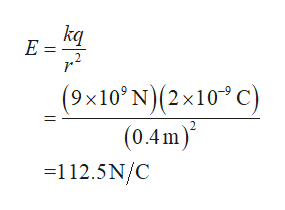a)A point charge of 2 × 10−9 C is located at the origin. What is the magnitude of the electric field at location ⟨0.4,0,0⟩ m?b) Next, a short, straight, thin copper wire 8 mm long is placed along the x-axis with its center at location ⟨0.2,0,0⟩ m. What is the approximate change in the magnitude of the electric field at location ⟨0.4,0,0⟩ m? (Approximate the polarized charges on the short copper wire as a dipole, and when calculating your answer, take the center of the wire as the point at which the net electric field is zero.)

Question

a)A point charge of 2 × 10−9 C is located at the origin. What is the magnitude of the electric field at location ⟨0.4,0,0⟩ m?

b) Next, a short, straight, thin copper wire 8 mm long is placed along the x-axis with its center at location ⟨0.2,0,0⟩ m. What is the approximate change in the magnitude of the electric field at location ⟨0.4,0,0⟩ m? (Approximate the polarized charges on the short copper wire as a dipole, and when calculating your answer, take the center of the wire as the point at which the net electric field is zero.)

Step 1

The below diagram represents the charge q that is placed at the origin, and a dipole placed on the x axis at a distance of 0.2m.

Step 2

(a)  Write the expression for electric field, and substitute 9×109 N for k,...help_outlineImage Transcriptionclosekq E = (9x10'N)(2x10 C) (0.4m) =112.5N/C 2 fullscreen

Want to see the full answer?

See Solution

Want to see this answer and more?

Our solutions are written by experts, many with advanced degrees, and available 24/7

See Solution
Tagged in

Physics